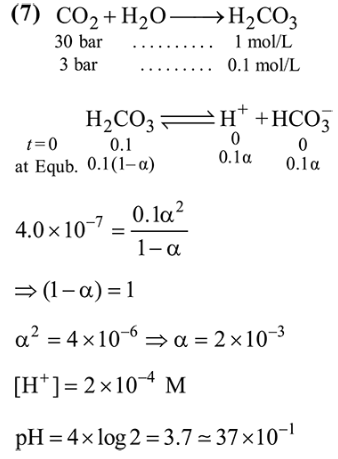# A soft drink was bottled with a partial prssure ofQuestion:

A soft drink was bottled with a partial prssure of $\mathrm{CO}_{2}$ of 3 bar over the liquid at room temperature. The partial pressure of $\mathrm{CO}_{2}$ over the solution approaches a value of 30 bar when $44 \mathrm{~g}$ of $\mathrm{CO}_{2}$ is dissolved in $1 \mathrm{~kg}$ of water at room temperature. The approximate $\mathrm{pH}$ of the soft drink is_____________ $\times 10^{-1}$.

(First dissociation constant of $\mathrm{H}_{2} \mathrm{CO}_{3}=4.0 \times 10^{-7} ; \log$ $2=0.3 ;$ density of the soft drink $=1 \mathrm{~g} \mathrm{~mL}^{-1}$ )

Solution: